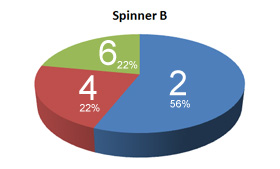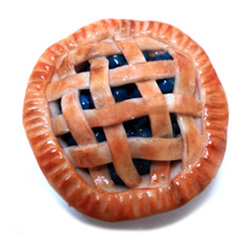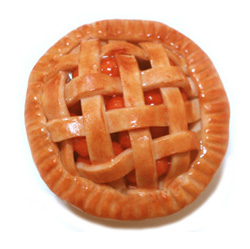CalGraph Bob's CalculusOnce upon a long time ago, before even video games existed, children played with cardboard spinners. The TV show, Wheel of Fortune, still uses a (somewhat fancier) spinner.A is boring. Anywhere the arrow lands, you get a "3".

B's arrow lands on "2" 56% of the time, "4" 22% of the time and "6" the remaining 22%.

C lands on "1" 51% of the time and "5" otherwise (49%).

You'll need to know a little bit about Probability to understand the rest.

#### Two-Kid Game

Which spinner would you choose if winning means getting the highest number?

In a competition between the first two spinners, as A always lands on "3", it wins only if B's arrow lands on "2".
P(A > B) = P(B = 2) = .56

A is a little better than C.
P(A > C) = P(C = 1) =.51

Poor C has even less chance with B.
P(B > C) = P( (B = 6) ∪ (C = 1) ) = P(B = 6) + P(C = 1) - P(B = 6)*P(C = 1) = .22 + .51 - .22 *.51 = .6178

Thus, in a 2-kid game, take A. If A is not available, choose B over C.

#### Three-Kid Game

Suppose that you have the first choice of spinner. After seeing the results for 2-kid games, honestly wouldn't you choose A, and if A was taken, reluctantly settle for B?

P(A wins) = P( (B = 2) ∩ (C = 1) ) = .56*.51 = .2851
Oops, A wins less than 1⁄3 of the time. This doesn't look good.

P(B wins) = P( (B = 6) ∪ ( (B = 4) ∩ (C = 1) ) ) = P(B = 6) + P(B = 4) * P(C = 1) = .22 + .22*.51= .3322
This is also less than 1⁄3. Since no ties are possible and we have found that A and B together win less than 2⁄3 of the time, it means that C must win more than 1/3 of the time. i.e. We already know that C is the best!

Just to check: P(C wins) = P(C = 5) * ( 1 - P(B = 6) ) = .51 * .78 = .3978

The hierarchy is reversed and therein the paradox!

A is best in 2-spinner competition, B second best and C the worst.
But, as we have just seen, in 3-spinner, C is the best, B the second best and A the worst.

How can we understand this?

Imagine there are 3 wrestlers, Al the Animal, Bombastic Bob and Charlie the Tuna. In a normal two person match, Al usually beats either Bob and Charlie. Bob wins more often than not against Charlie.
In a "Last Man Standing" (3 person match), Al and Bob each think that the other is the greater threat so they ignore Charlie and knock each other out. Charlie wins!

In fact, there was a year in which the champion at the end of the season of the World Cup of Skiing didn't win a single race. The idea is that the most daring skiers A and B fall down trying to beat each other. Then the safe and not so sorry skier C wins by default.

#### A Mathematician's Story

I eat lunch in a greasy spoon. (What else can a teacher afford?)
They have three desserts.Normally only two of them are available.
Apple pie is always mediocre. (Sorry, Americans) On a scale of 1 to 6, I think it merits a "3".
When blueberry pie is made from jam, rather than fresh blueberry, as it is 56% of the time, I give it a "2" rating. I rate it a "4" or "6" each about 22% of the time depending on if the blueberries are fresh.
Cherry pie is the pits slightly more than half the time ("1" rating) and otherwise almost marvelous ("5" rating).

One morning, the cook stays sober long enough to make all three pies. The waiter, who has gotten to know my preferences, says to me, "Since you always pick apple over blueberry, apple over cherry and blueberry over cherry, I guess you'll take apple today."

The waiter will have to visit this webpage to understand why, when three pies are available, the Mathematician chooses cherry!

#### Footnotes

This bit of Mathematical confusion was discovered by a possibly sadistic Marquis known as Condorcet.

At political conventions, it has happened that a candidate other than one of the two most popular has won. e.g. Joe Clark's 1976 leadership win.

All the arithmetic was checked with CalGraph.

## Back to Fun Stuff